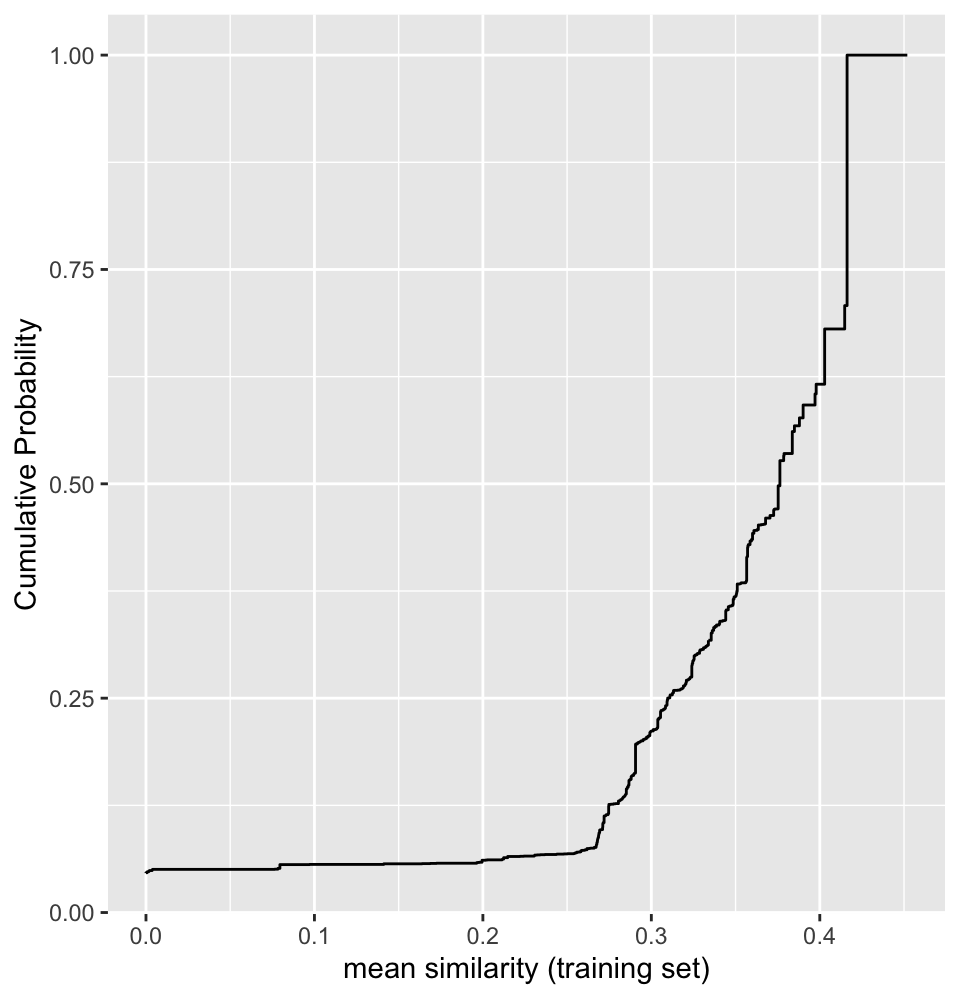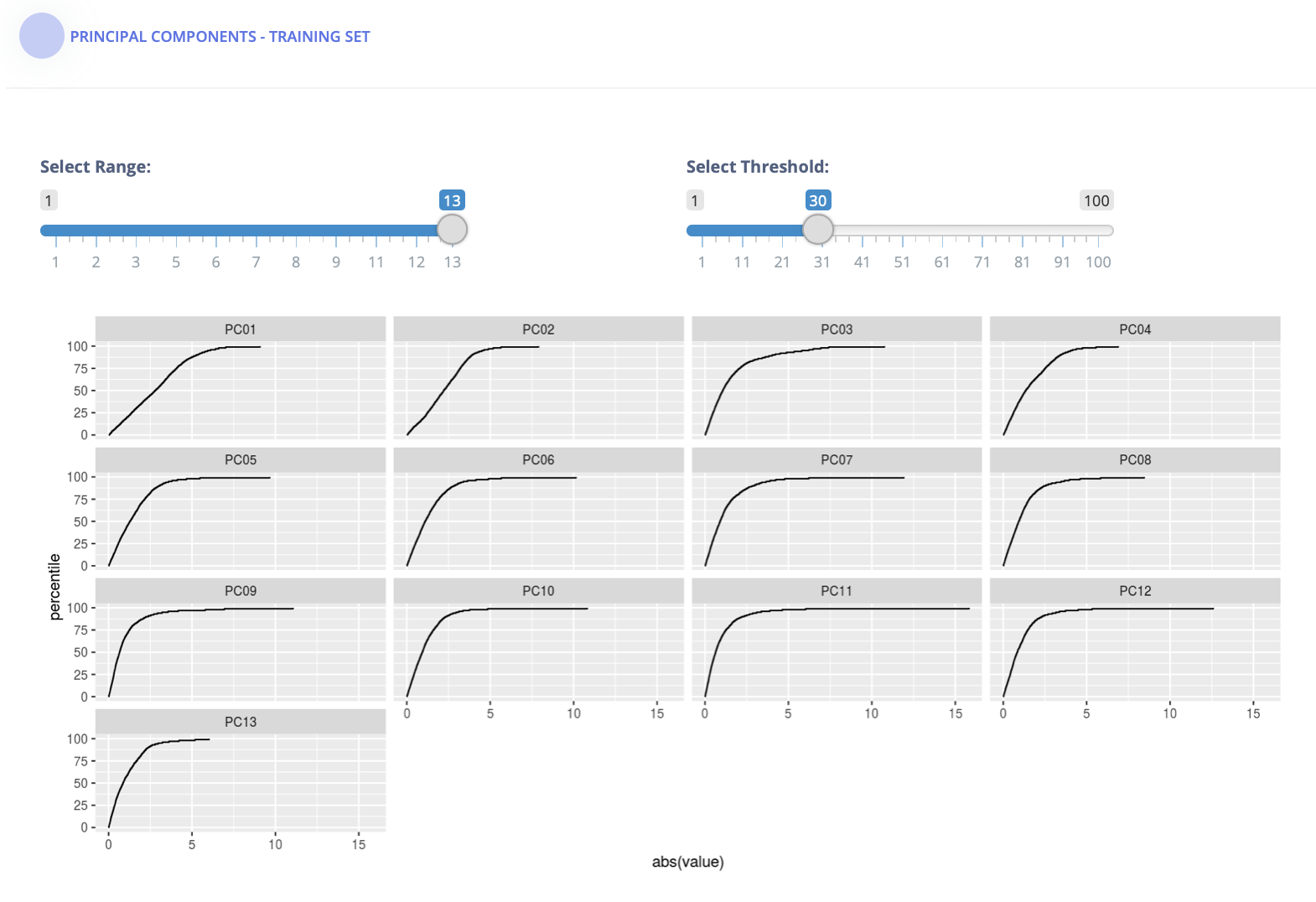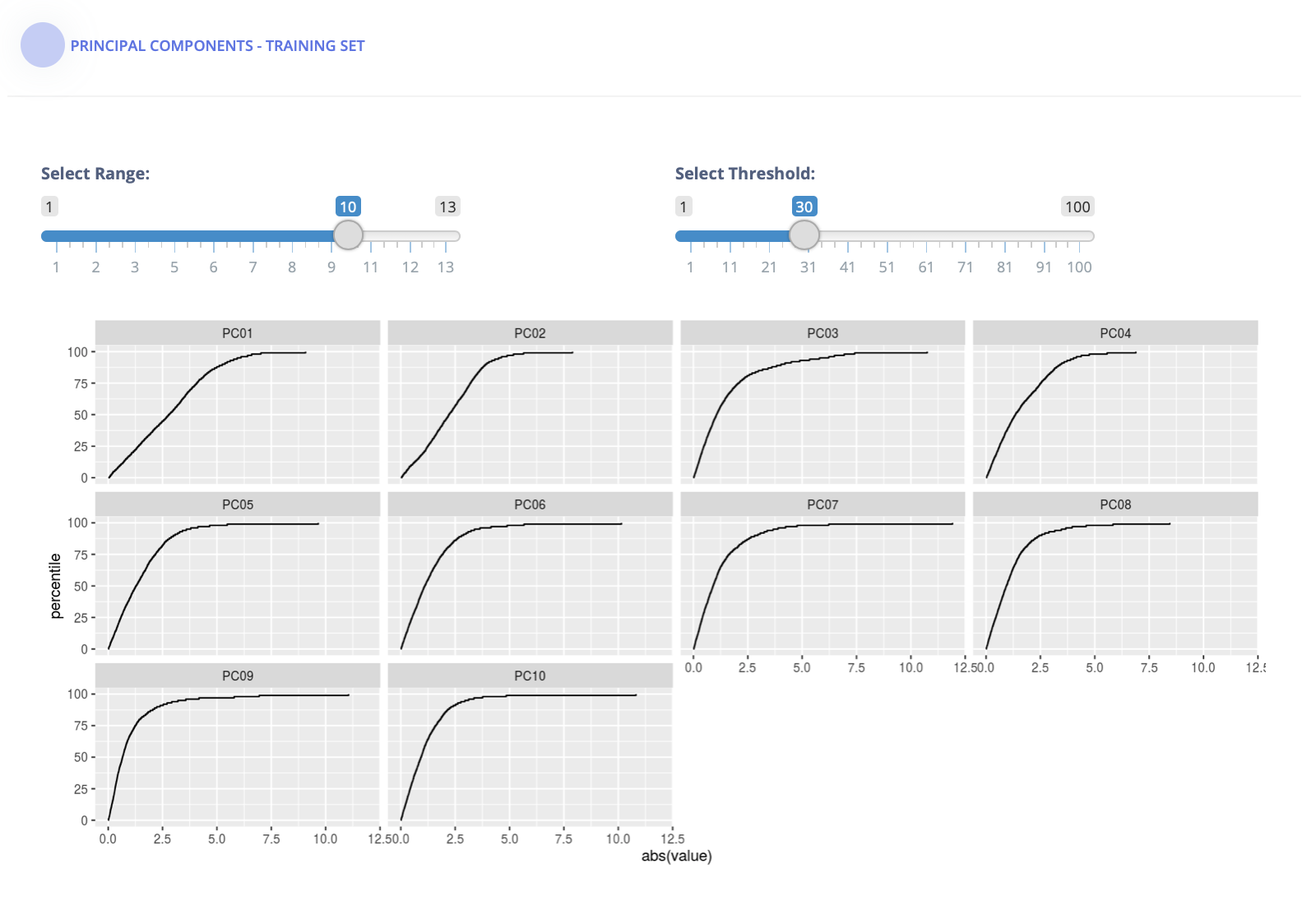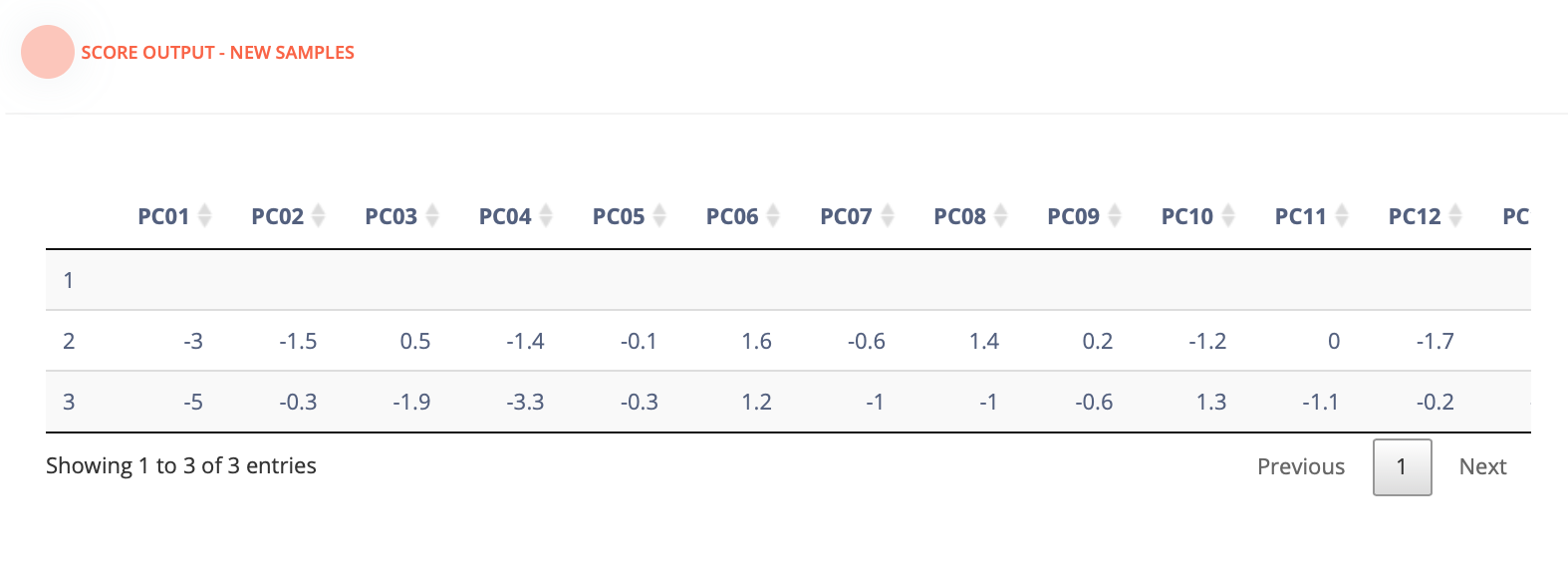# This Is Not Like the Others

This summer I had the opportunity of working as an intern for RStudio. Among the available projects/mentors, I was fortunate to collaborate with Max Kuhn developing the applicable modeling R package now available here. The package comprises of a collection of applicability domain models to measure the reliability of a given model’s prediction. These models are borrowed from Chemistry, where they are widely used to measure the reliability of QSARs models.

## Applicability Domain Methods

Applicability domain (AD) models are unsupervised approaches to characterize the nature of a prediction’s extrapolation. They are based on the premise that a model’s prediction is reliable if the sample from which a prediction is made is similar to the training set. The following are examples of AD methods:

• Simple distance measures can be used, e.g., the leverage or hat value of a data point.

• Similarity measurements, which are preferred when the predictor space consists of all binary predictors. A common metric is the Jaccard index.

Let us dive into some of these methods through the applicable package.

## The applicable package

The applicable package contains models to analyze binary and continuous data. The Jaccard index – a similarity statistics – is a specialized indicator when working with binary data. On the other hand, for continuous data, both principal component analysis and hat values are employed.

library(applicable)


## Binary data: the Jaccard index

Similarity statistics can be used to compare data sets where all of the predictors are binary. One of the most common measures is the Jaccard index. Given sets A and B, the Jaccard similarity between A and B is defined as

$$J(A, B) = \frac{\|A \cap B\|}{\|A \cup B\|}$$

For a training set of size n, there are n similarity statistics for each new sample. These can be summarized via the mean statistic or a quantile. In general, we want similarity to be low within the training set (i.e., a diverse training set) and high for new samples to be predicted.

To analyze the Jaccard metric, applicable provides the following methods:

• apd_similarity: analyzes samples in terms of similarity scores. For a training set of n samples, a new sample is compared to each, resulting in n similarity scores. These can be summarized into the median similarity.

• autoplot: shows the cumulative probability versus the unique similarity values in the training set.

• score: scores new samples using similarity methods. In particular, it calculates the similarity scores and if add_percentile = TRUE, it also estimates the percentile of the similarity scores.

The example data is from two QSAR data sets where binary fingerprints are used as predictors.

data(qsar_binary)


Let us construct the model:

jacc_sim <- apd_similarity(binary_tr)
jacc_sim

## Applicability domain via similarity
## Reference data were 67 variables collected on 4330 data points.
## New data summarized using the mean.


As we can see below, this is a fairly diverse training set:

library(ggplot2)

# Plot the empirical cumulative distribution function for the training set
autoplot(jacc_sim)We can compare the similarity between new samples and the training set:

# Summarize across all training set similarities
mean_sim <- score(jacc_sim, new_data = binary_unk)
mean_sim

## # A tibble: 5 x 2
##   similarity similarity_pctl
##        <dbl>           <dbl>
## 1     0.376            49.8
## 2     0.284            13.5
## 3     0.218             6.46
## 4     0.452           100
## 5     0.0971            5.59


Samples 3 and 5 are definitely extrapolations based on these predictors. In other words, the new samples are not similar to the training set and so predictions on them may not be very reliable.

library(applicable)


## Continuous data: PCA

When working with continuous data, applicable provides the following methods to analyze the applicability domain of your model:

• Principal component analysis
• Hat values statistics

To see applicable in action, let us look at the principal component analysis of the Ames IA housing data.

library(AmesHousing)
ames <- make_ames()


There are 2,930 properties in the data.

The Sale Price was recorded along with 81 predictors, including:

• Location (e.g., neighborhood) and lot information.
• House components (garage, fireplace, pool, porch, etc.).
• General assessments such as overall quality and condition.
• Number of bedrooms, baths, and so on.

More details can be found in De Cock (2011, Journal of Statistics Education).

The raw data are at http://bit.ly/2whgsQM but we will use a processed version found in the AmesHousing package. applicable also contains an update for these data for three new properties (although fewer fields were collected on these).

To pre-process the training set, we will use the recipes package. We first tell the recipes that there is an additional value for the neighborhood in these data, then direct it to create dummy variables for all categorical predictors. In cases where there are no levels observed for a factor, we eliminate predictors with a single unique value, then estimate a transformation that will make the predictor distributions more symmetric. After these, the data are centered and scaled. These same transformations will be applied to the new data points using the statistics estimated from the training set.

library(recipes)
library(dplyr)

ames_cols <- names(ames_new)
ames_new$Neighborhood <- as.factor(ames_new$Neighborhood)

training_data <-
ames %>%
# For consistency, only analyze the data on new properties
dplyr::select(one_of(ames_cols)) %>%
mutate(
# There is a new neighborhood in ames_new
Neighborhood = as.character(Neighborhood),
Neighborhood = factor(Neighborhood, levels = levels(ames_new\$Neighborhood))
)

training_recipe <-
recipe( ~ ., data = training_data) %>%
step_dummy(all_nominal()) %>%
# Remove variables that have the same value for every data point.
step_zv(all_predictors()) %>%
# Transform variables to be distributed as Gaussian-like as possible.
step_YeoJohnson(all_numeric()) %>%
# Normalize numeric data to have a mean of zero and
# standard deviation of one.
step_normalize(all_numeric())


The following functions in applicable are used for principal component analysis:

• apd_pca: computes the principal components that account for up to either 95% or the provided threshold of variability. It also computes the percentiles of the principal components and the mean of each principal component.
• autoplot: plots the distribution function for pcas. You can also provide an optional set of dplyr selectors, such as dplyr::matches() or dplyr::starts_with(), for selecting which variables should be shown in the plot.
• score: calculates the principal components of the new data and their percentiles as compared to the training data. The number of principal components computed depends on the threshold given at fit time. It also computes the multivariate distance between each principal component and its mean.

To see how these functions work, we will borrow the outputs of the shiny application we are currently developing for the package.

Let us apply apd_pca modeling function to our data:

ames_pca <- apd_pca(training_recipe, training_data)


Since no threshold was provided, the function computed the number of principal components that accounted for at most 95% of the total variance. Plotting the distribution function for the PCA scores is also helpful. In the shiny application, we can set the threshold as well as the subset of PCAs displayed.

For illustration, setting threshold = 0.30 or 30%, we now need only 13 principal components:We can also update the number of PCAs displayed:Finally, the score function compares the training data to new samples. This is the output of pca_score <- score(ames_pca, ames_new):## Conclusion

The project was ambitious and complex in nature, which made it that much more intereting. I learned a lot from Max and had an amazing experience working for RStudio. As I mentioned before, an application using the shiny package is currently in development. You can take a pick at it here.

Contents